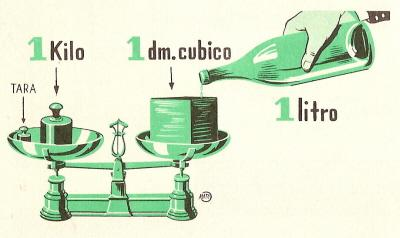Units of mass (weight) to printName: ______________________________________  Subject: _______________________ Date: _______

Write on the right side what is missing.

1. The gram.

A liter of water weighs 1 kilogram or 1000 grams. The unit of measurement of mass (weight) is the gram and its abbreviation is g.

2. Multiples of the gram

These are:

1 decagram is equal to 10 grams: 1 dag = 10 g.
1 hectogram is equal to 100 grams: 1 hg = 100 g.
1 kilogram is equal to 1000 grams: 1 kg = 1000 g.
1 myriagram is equal to 10000 grams: 1 myg = 10000 g.
1 hundredweight is equal to 100 kilograms: 1 cwt = 100 kg.
1 tonne is equal to 1000 kilograms: 1 t = 1000 kg.

It answers these questions in grams:

 3 hg = 7 kg = 6 dag =

3. Submultiples of the gram.

These are:

1 decigram is equal to 0.1 grams: 1 dg = 0.1 g. The gram has 10 decigrams.
1 centigram is equal to 0.01 grams: 1 cg = 0.01 g. The gram has 100 centigrams.
1 milligram is equal to 0.001 gram: 1 mg = 0.001 g. The gram has 1000 milligrams.

 5 cg = 7 mg = 3 dg=

4. Conversion of unit.

Each unit of mass (weight) is 10 times greater than the immediate lower and 10 times less than the immediate superior.

To convert from hg to dag, we multiply by 10 or we move the decimal point one place to the right.
Examples: 7 hg = 70 dag; 237,25 g = 2372,5 dg = 23725 cg.

To convert from g to dag, we divide by 10 or we move the decimal point one place to the left.
Examples: 60 g = 6 dag; 1468 g = 146,8 dag = 14,68 hg = 1.468 kg.

 9 dag = 7,3 kg = 265 mg =

5. Converting from complex to non-complex

To convert from complex number to non-complex number of lower order, we must write the numbers that represent the units of the various orders from left to right, and one after the other. We have to start with the greatest order. If it lacks some order, we must put a zero in the appropriate place.

Example: 4 kg, 6 dag and 7 g can be written as: 4067 g.

 17 hg, 3 g y 7 cg 4 kg, 2 dag y 4 mg = 9 mag, 3 g y 2 cg =

6. Converting from non-complex (74 g ) to complex (7 dag and 4 g)

To convert from non-complex number to a complex number, we have to take into account that the number of units has the same order as the non-complex, the tens of the immediate superior order and so on. If there are zeros in the number, we avoid the zero and skip the order that corresponds to it.

Example: 10203.045 g = 1 mg, 2 hg, 3 g, 4 cg and 5 mg.

Answers in non-complex number:

 3,07 g = 403 g = 80,7 g =

7. Problems.

Do these problems on paper and write the solution:

 1. If a bag of candies is 125 g. How many parcels of the same weight can I form with 5 kg of candies? 2. A man sells 143 liters of wine from a barrel that contains 300 liters. He spills 7 liters. How many liters does he have? 3. Find the difference in meters between two paths, if one is 7 km, 5 dam and 3 m long and another is 26 hm and 6 m. 4. A chocolate is 8 grams. How many hectograms is 200 chocolates? 5. A jar contains 4 hl of oil and it cost 1000 euros. How much does the liter cost? 6. We have to fix a stretch of road that is 30 km. we have already repaired 6321 m. How many meters do we have to repair to finish? 7. A box contains 120 apples. If the average weight of an apple is 75 g. How many kg will all the apples weigh? 8. A vintner buys 20 hl of wine. First he sold 120 liters and he distributes the rest in 8 equal barrels. How many liters does he have placed in each barrel? 9. The water tank is 3 km and 6 hm from the village. How many pipes of half decimeter long do we need to bring the water to the village? 10. A ship transports 2800 tons of goods. How many carriages will be required to transport the goods if each carriage loads 1400 kg?

| Educational applications | Mathematics |
In Spanish  | Interactive

«Arturo Ramo GarcĒa.-Record of intellectual property of Teruel (Spain) No 141, of 29-IX-1999
Plaza Playa de Aro, 3, 1║ DO 44002-TERUEL